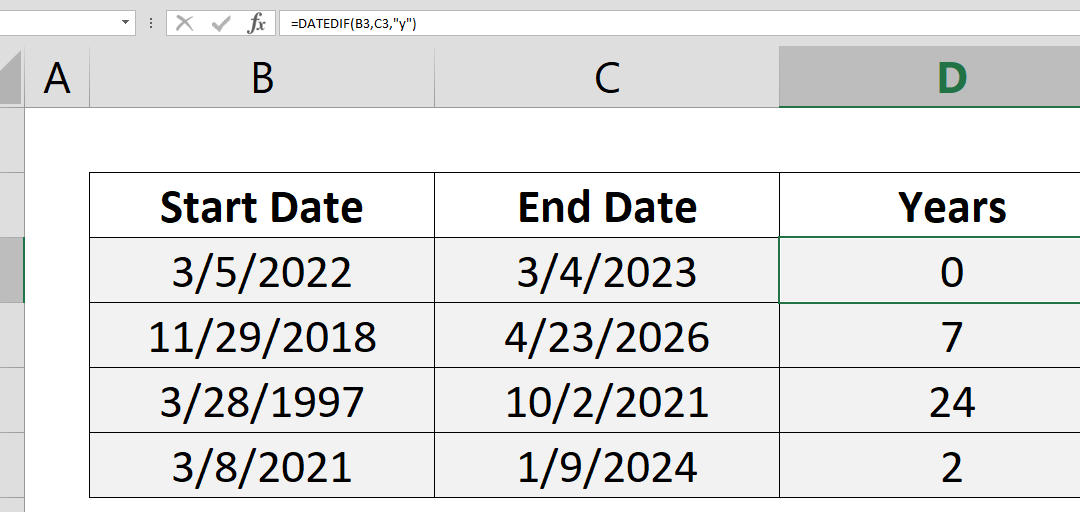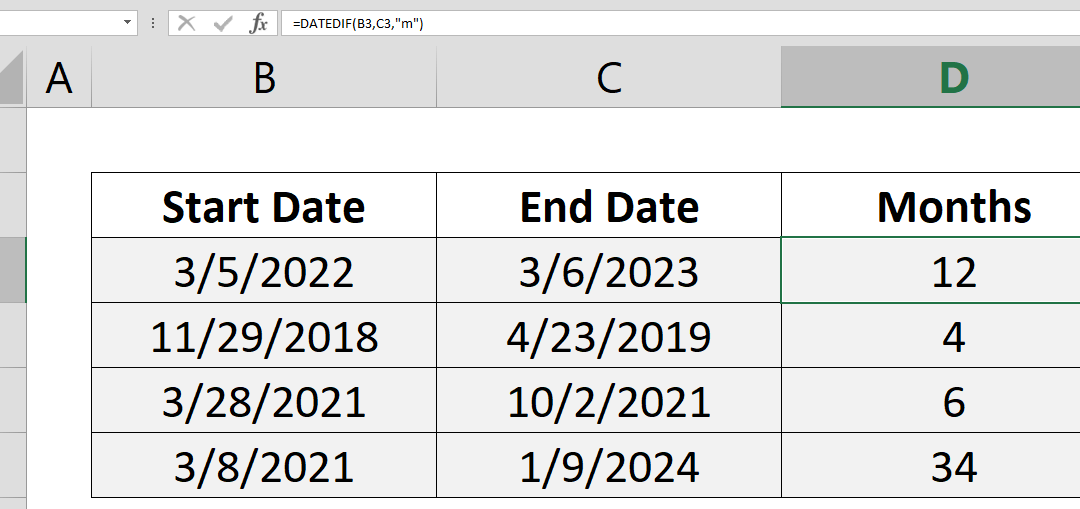Select Page## How to find nth smallest value in a data table

This article shows you how to find nth smallest value in a data table by using the SMALL function. Syntax =SMALL(absolute data range reference, nth) Steps Start with =SMALL( function Select the range that contains the values \$B\$2:\$G\$7, Select the cell that contains...## How to find nth largest value in a data table

This article shows you that how to find nth largest value by using the LARGE function. Syntax =LARGE(absolute data range reference, nth) Steps Start with =LARGE( function Select the range that contains the values \$B\$2:\$G\$7, Select the cell that contains nth value or...## How to highlight cells by values

Highlighting cells is a good way to visualize content to locate areas that needs attention. Excel’s Conditional Formatting feature is a great tool for this job. How to highlight cells by values article will show how you can create highlight cells based on...## How to count values by length

This article shows how to count values by length based on their character count. This method can be helpful to check your data against invalid entries. It is also a good practice to use SUMPRODUCT and N functions together. Syntax =SUMPRODUCT(N(LEN(range)>character...## How to count years between two dates with the DATEDIF

This article show you how to count years between two dates using the DATEDIF function. Syntax =DATEDIF(start date, end date, “y”) Steps Use =DATEDIF( Select or type range reference that includes start date B3, Select or type range reference that includes...## How to count months between two dates with the DATEDIF

This article show you how to count months between two dates using DATEDIF function. Syntax =DATEDIF(start date, end date, “m”) Steps Use =DATEDIF( Select or type range reference that includes start date B3, Select or type range reference that includes end...## How to SUM 2d ranges with SUMPRODUCT

Summing values by a condition may sound easy. If not, please see Crunch Data Even Faster with the SUMIFS Function article as well. You can easily sum values by condition(s) with SUMIF and SUMIFS functions; with a limitation. The sum range and criteria ranges should...## How to SUM values across sheets

To sum range of cells in the same worksheet is one of the basic concepts of Excel. What about summing values across sheets? In this article we will explain how to sum values across sheets. Syntax =SUM(first sheet : second sheet ! reference) Steps Start with =SUM( Type...## How to count values if date is less than by using COUNTIF function

Using the versatile COUNTIF and COUNTIFS functions, see how you can count cells up to a certain date. How to count values if date is less than by using COUNTIF function article shows an essential tip for creating YTD (year-to-date) reports. Syntax =COUNTIFS(date...